1.線性迴歸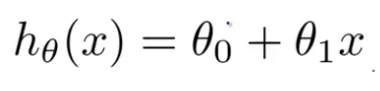Note:我這裡用w代表theta，他的實際含義都是“weight”,建議相關的知識細節，到這裡學習！！！

2.成本函式（cost function）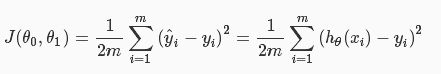3.引數學習（梯度下降）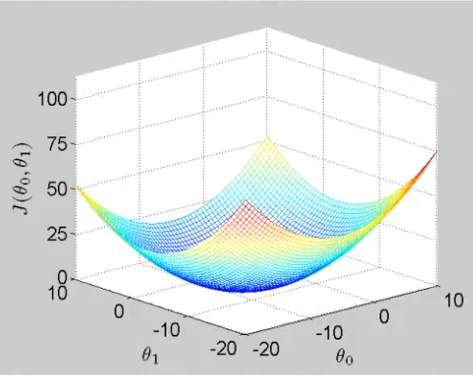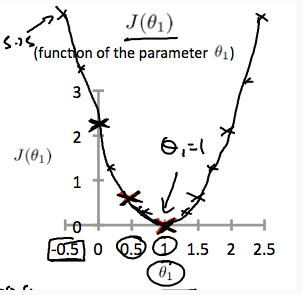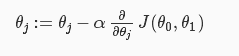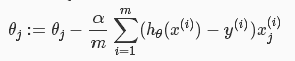4.線性迴歸模型Python程式碼實現

print diabetes.keys()
data = diabetes.data #real -0.2<x<0.2
target = diabetes.target #integer 25<y<346
print data.shape
print target.shape
print data[:5]
print target[:5]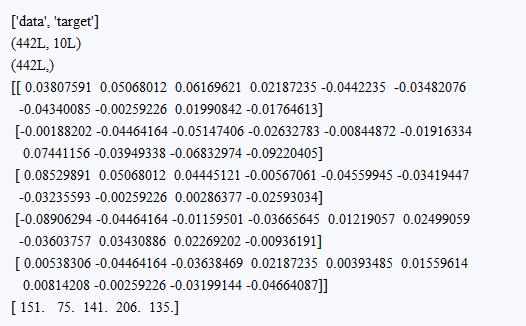import numpy as np
#use only one feature
X = data[:,:1]
y = target
#X_train = np.array([, , ])
#X_test = np.array([, , ])
#y_train = np.array([0,1,2]).reshape(-1,1)
#y_test = np.array([3,4,5]).reshape(-1,1)
X_train = X[:-20]
X_test = X[-20:]
y_train = y[:-20].reshape((-1,1))
y_test = y[-20:].reshape((-1,1))
print 'X_train=',X_train.shape
print 'X_test=',X_test.shape
print 'y_train=',y_train.shape
print 'y_test=',y_test.shape

class linear(object):
def __init__(self):
self.W = None
self.b = None

def loss(self,X,y):
num_feature  = X.shape
num_train = X.shape

h = X.dot(self.W) + self.b
loss = 0.5 *np.sum(np.square(h - y)) / num_train

dW = X.T.dot((h-y)) / num_train
db = np.sum((h-y)) / num_train

return loss,dW,db

def train(self,X,y,learn_rate = 0.001,iters = 10000):
num_feature = X.shape
self.W = np.zeros((num_feature,1))
self.b = 0
loss_list = []

for i in xrange(iters):
loss,dW,db = self.loss(X,y)
loss_list.append(loss)
self.W += -learn_rate*dW
self.b += -learn_rate*db

if i%500 == 0:
print 'iters = %d,loss = %f' % (i,loss)
return loss_list

def predict(self,X_test):
y_pred = X.dot(self.W) + self.b
return y_pred

pass

classify = linear()
print 'start'
loss_list = classify.train(X_train,y_train)
print 'end'

print classify.W,classify.b

import matplotlib.pyplot as plt
f = X_train.dot(classify.W) + classify.b
fig = plt.figure()
plt.subplot(211)
plt.scatter(X_train,y_train,color = 'black')
plt.scatter(X_test,y_test,color = 'blue')
plt.plot(X_train,f,color = 'red')
plt.xlabel('X')
plt.ylabel('y')

plt.subplot(212)
plt.plot(loss_list,color = 'blue')
plt.xlabel('epochs')
plt.ylabel('errors')
plt.show()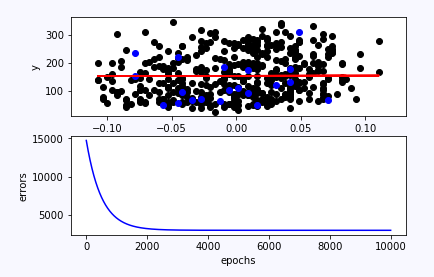ps:至於其他的一些細節：正則化、特徵歸一化、多項式特徵等問題，等有空再上程式碼，畢竟一下子也說不清。